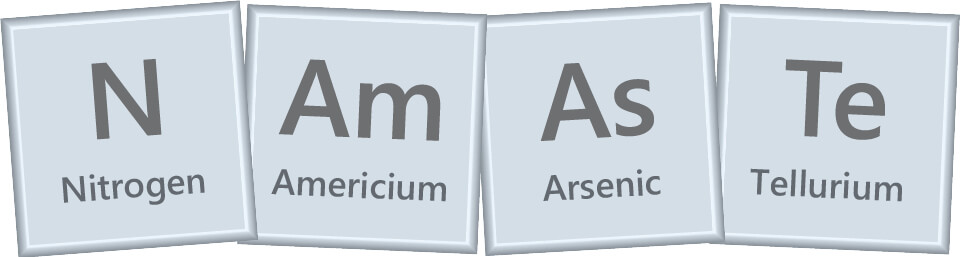Learning Objective

In this lesson we will learn how to write and interpret chemical formulas for elements and compounds.

Learning Outcomes

By the end of this lesson you will be able to:

• Write chemical symbols for elements.
• Write chemical formulas for compounds.
• Identify the number of each type of atom in a molecule based on its chemical formula.
• Distinguish between elements and compounds based on their chemical symbols/formulas.

Names and Symbols for Elements and Compounds

1 | Symbols for Elements

2 | Formulas for Compounds

3 | Some Things to Remember

4 | Summary

Click images to preview the worksheet for this lesson and the Year 8 Chemistry Workbook (PDF and print versions)

## Introduction

• The names of atoms and elements can be represented by abbreviated symbols, which can be combined into formulas to represent molecules and compounds.
• These chemical symbols and formulas provide a quick and universal way of identifying pure substances in chemistry.

## Symbols for Elements

• Each type of atom or element can be represented by a unique chemical symbol consisting of one or two letters.
• The first letter is written as a capital and the second letter, if there is one, is written in lowercase.
• Examples
• ▸ Carbon can be represented by the symbol C.
• ▸ Neon can be represented by the symbol Ne.
• ▸ Silver can be represented by the symbol Ag.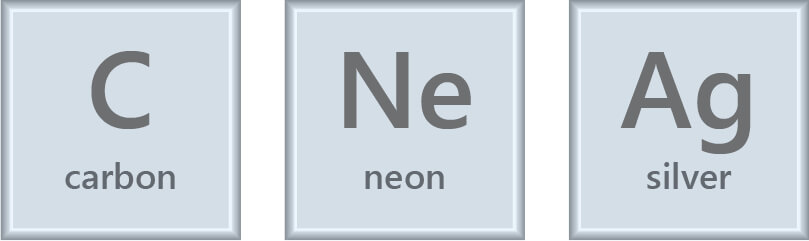• Chemical symbols are abbreviations for the name of the element.
• Often the symbols are based on the Latin name for the element, so it isn’t always obvious why an element has a particular symbol.
• Example 1
• The symbol “C” for carbon is based on the Latin word “carbo”, meaning “coal”.
• This is quite similar to the English name.
• Example 2
• The symbol “Ag” for silver is based on the Latin word “argentum”, meaning “shiny”.
• This is very different to the English name.
• It’s not necessary to memorise the chemical symbols of all the elements (as you can always consult a periodic table), but it can be helpful to memorise the common ones.
• For example, try learning the names and symbols of the first 20 elements, as you will encounter these a lot in chemistry. They are listed in the following table.

•  Element Number Element Name Element Symbol 1 Hydrogen H 2 Helium He 3 Lithium Li 4 Beryllium Be 5 Boron B 6 Carbon C 7 Nitrogen N 8 Oxygen O 9 Fluorine F 10 Neon Ne 11 Sodium Na 12 Magnesium Mg 13 Aluminium Al 14 Silicon Si 15 Phosphorus P 16 Sulfur S 17 Chlorine Cl 18 Argon Ar 19 Potassium K 20 Calcium Ca

The names and symbols of the first 20 elements.

• Commonly encountered metal elements outside of the first 20 are shown in the following table.

•  Element Name Element Symbol Iron Fe Copper Cu Zinc Zn Silver Ag Tin Sn Gold Au Lead Pb

Common metals outside of the first 20 elements.

• Commonly encountered non-metal elements outside of the first 20 are shown in the following table.

•  Element Name Element Symbol Bromine Br Iodine I

Common non-metals outside of the first 20 elements.

## Formulas for Compounds

• Each type of molecule or compound can also be represented by a chemical formula.
• The formula for a compound represents the number of each type of atom in its molecules or subunits.
• Chemical formulas consist of two parts:
• Chemical symbols of each type of atom.
• Numbers (subscripts) indicating how many of each atom is in a molecule or subunit (if there is more than one).
• Example 1
• Water can be represented by the chemical formula H2O.
• The symbols H and O show that a water molecule is made up of hydrogen atoms and
oxygen atoms.
• (We could also say that water is formed from the elements hydrogen and oxygen.)
• The subscript 2 after the H indicates there are two hydrogen atoms in a water molecule.
• No subscript after the O indicates there is one oxygen atom in a water molecule.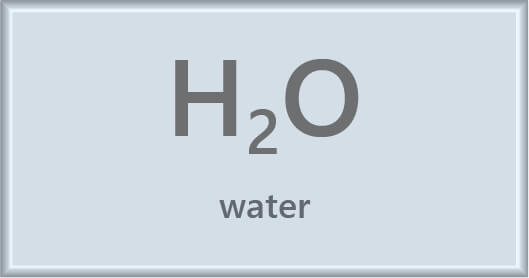• Example 2
• Calcium hydroxide can be represented by the chemical formula Ca(OH)2.
• The symbols Ca, O and H show that calcium hydroxide is composed of calcium, oxygen and hydrogen atoms.
• (We could also say that calcium hydroxide is formed from the elements calcium, oxygen and hydrogen.)
• No subscript after the Ca indicates there is one calcium atom in a calcium hydroxide molecule.
• The subscript 2 indicates there is two of everything inside the brackets.
• Therefore, there are two oxygen atoms and two hydrogen atoms in a calcium hydroxide molecule.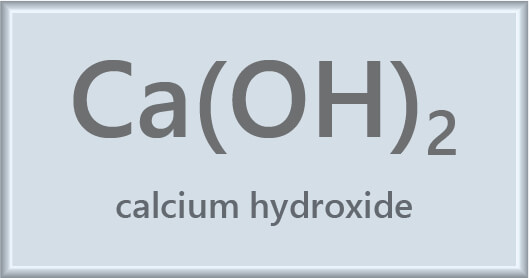• Sometimes the name of a compound can give a clue its formula.
• For example, the name carbon dioxide suggests that this compound consists of molecules containing one carbon atom and two oxygen atoms (“di” means two).
• However, the names for most compounds, such as methane, ethanol and glucose, don’t provide enough information to determine their formulas.
• As with elements, it can be useful to memorise the names and formulas of common compounds.
• Some compounds that you will encounter regularly in junior chemistry are shown in the following table.

•  Compound Name Compound Formula Water H2O Carbon dioxide CO2 Carbon monoxide CO Hydrochloric acid HCl Sulfuric acid H2SO4 Nitric acid HNO3 Sodium hydroxide NaOH Ammonia NH3 Sodium chloride NaCl Copper sulfate CuSO4 Calcium carbonate CaCO3

The names and formulas of some common compounds.

## Some Things to Remember

• Many students get confused when writing the symbols for elements that exist as molecules, such as hydrogen, oxygen and chlorine.
• The correct symbol depends on whether you are writing it to represent the atom or the element (the actual substance), which is made up of molecules.
• For example, the symbol for an oxygen atom is O, but the symbol for the element oxygen (oxygen gas) is O2.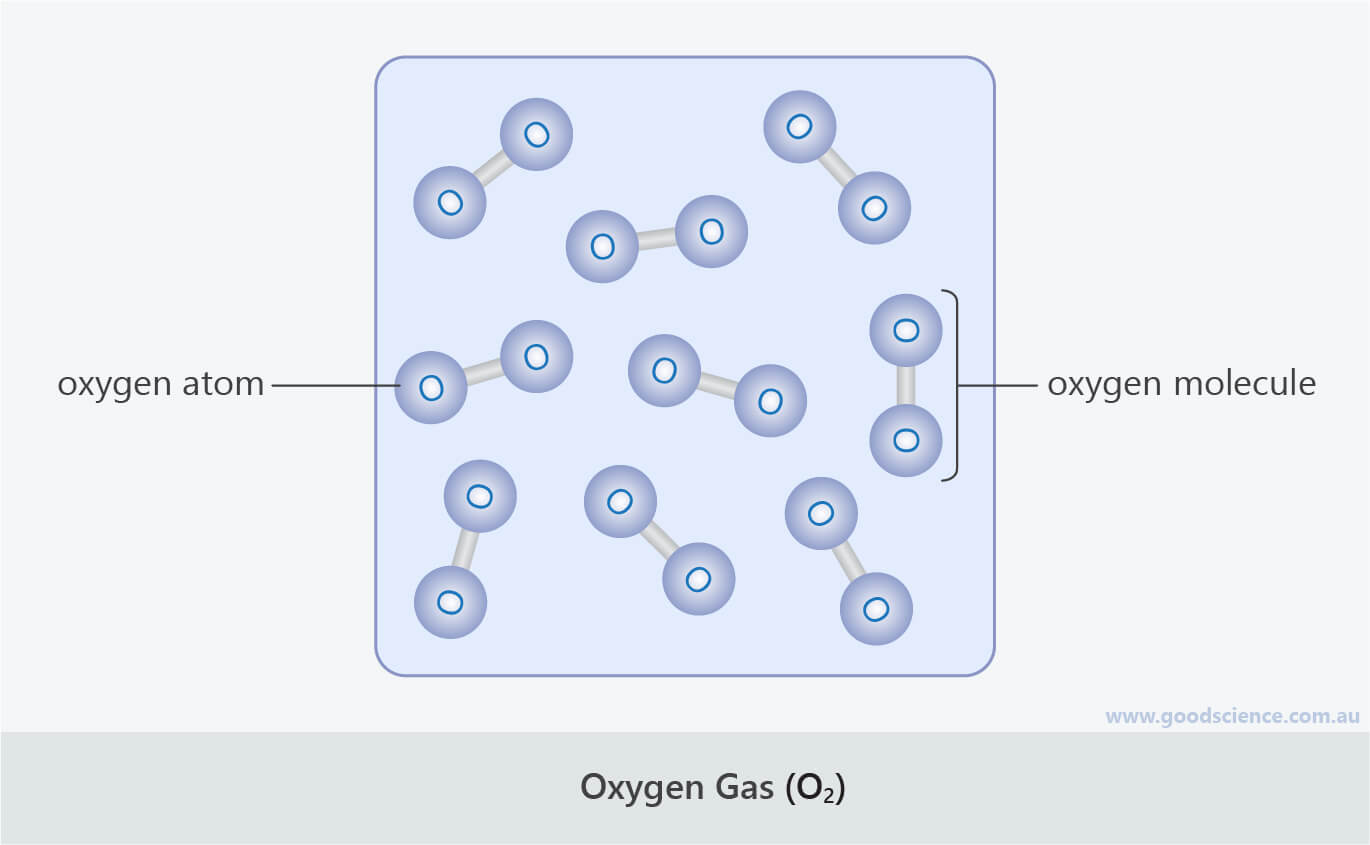Oxygen atoms have the symbol “O”, but since oxygen gas is made up of molecules containing two oxygen atoms, its symbol is “O2“.

• Remember that symbols for elements consist of only one capital letter.
• For example, there is a big difference between Co and CO. One is a metal element and one is a compound formed from two non-metal elements.
• By remembering this, you can also quickly determine if a substance is an element or a compound by checking if it contains one or more than one capital letter in its formula.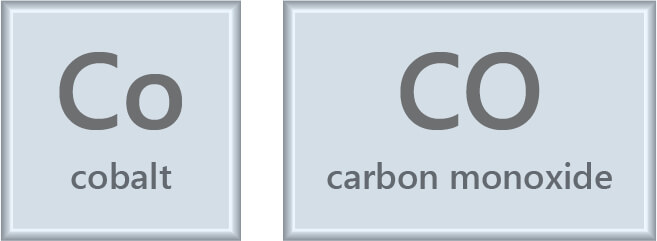Lowercase or capital letter? It makes a big difference in chemistry!
“Co” is the symbol for the element cobalt, which is a solid metal.
“CO” is the symbol for the compound carbon monoxide, which is a colourless gas.

• When counting up the total number of a type of atom in a compound, check if the element symbol is present more than once.
• For example, the formula for acetic acid (CH3COOH) has carbon, hydrogen and oxygen in more than one place. (It is written like this to reflect the structure of the molecule).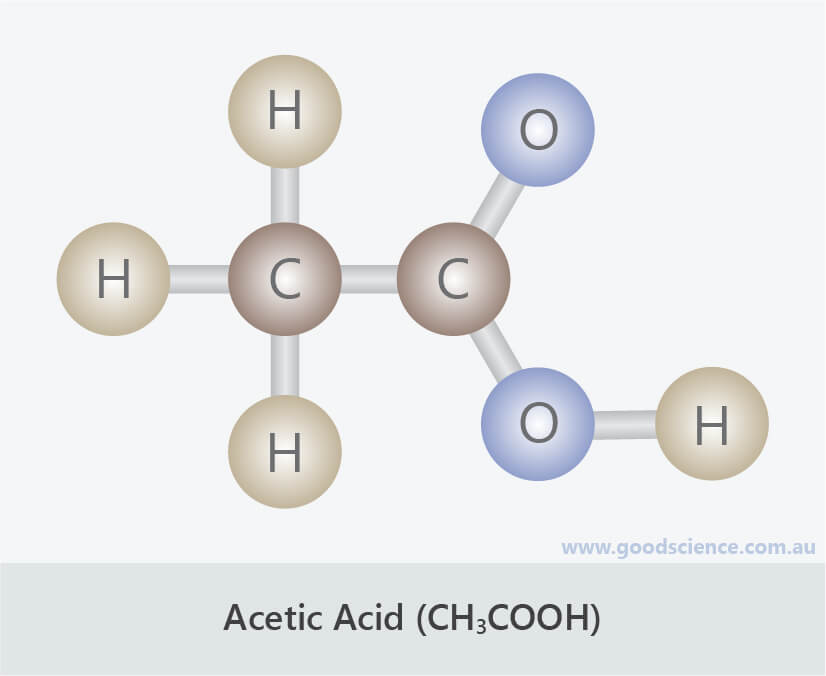Sometimes the same type of atom can appear in more than one place in a molecular formula.

## Summary

• Each type of atom or element can be represented by a unique chemical symbol consisting of one or two letters.
• The first letter is a capital and the second letter, if there is one, is lowercase.
• Each type of molecule or compound can be represented by a chemical formula.
• The formula represents the number of each type of atom in a molecule.
• It is therefore a combination of the symbols for the different atoms and numbers (subscripts) indicating how many of each atom is in a molecule.